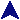Polynomial EvaluatorRuby Example Code#
# This program evaluates polynomials.  It first asks for the coefficients
# of a polynomial, which must be entered on one line, highest-order first.
# It then requests values of x and will compute the value of the poly for
# each x.  It will repeatly ask for x values, unless you the user enters
# a blank line.  It that case, it will ask for another polynomial.  If the
# user types quit for either input, the program immediately exits.
#

#
# Function to evaluate a polynomial at x.  The polynomial is given
# as a list of coefficients, from the greatest to the least.
def polyval(x, coef)
sum = 0
coef = coef.clone           # Don't want to destroy the original
while true
sum += coef.shift       # Add and remove the next coef
break if coef.empty?    # If no more, done entirely.
sum *= x                # This happens the right number of times.
end
return sum
end

#
# Function to read a line containing a list of integers and return
# them as an array of integers.  If the string conversion fails, it
# throws TypeError.  If the input line is the word 'quit', then it
# converts it to an end-of-file exception
print prompt
raise EOFError.new if line == 'quit' # You can also use a real EOF.

# Go through each item on the line, converting each one and adding it
# to retval.
retval = [ ]
for str in line.split(/\s+/)
if str =~ /^\-?\d+\$/
retval.push(str.to_i)
else
raise TypeError.new
end
end

return retval
end

#
# Take a coeff and an exponent and return the string representation, ignoring
# the sign of the coefficient.
def term_to_str(coef, exp)
ret = ""

# Show coeff, unless it's 1 or at the right
coef = coef.abs
ret = coef.to_s     unless coef == 1 && exp > 0
ret += "x" if exp > 0                               # x if exponent not 0
ret += "^" + exp.to_s if exp > 1                    # ^exponent, if > 1.

return ret
end

#
# Create a string of the polynomial in sort-of-readable form.
def polystr(p)
# Get the exponent of first coefficient, plus 1.
exp = p.length

# Assign exponents to each term, making pairs of coeff and exponent,
# Then get rid of the zero terms.
p = (p.map { |c| exp -= 1; [ c, exp ] }).select { |p| p != 0 }

# If there's nothing left, it's a zero
return "0" if p.empty?

# *** Now p is a non-empty list of [ coef, exponent ] pairs. ***

# Convert the first term, preceded by a "-" if it's negative.
result = (if p < 0 then "-" else "" end) + term_to_str(*p)

# Convert the rest of the terms, in each case adding the appropriate
# + or - separating them.
for term in p[1...p.length]
# Add the separator then the rep. of the term.
result += (if term < 0 then " - " else " + " end) +
term_to_str(*term)
end

return result
end

#
# Run until some kind of endfile.
begin
# Repeat until an exception or quit gets us out.
while true
# Read a poly until it works.  An EOF will except out of the
# program.
print "\n"
begin
poly = readints("Enter a polynomial coefficients: ")
rescue TypeError
print "Try again.\n"
retry
end
break if poly.empty?

# Read and evaluate x values until the user types a blank line.
# Again, an EOF will except out of the pgm.
while true
# Request an integer.
print "Enter x value or blank line: "
break if x == ''
raise EOFError.new if x == 'quit'

# If it looks bad, let's try again.
if x !~ /^\-?\d+\$/
print "That doesn't look like an integer.  Please try again.\n"
next
end

# Convert to an integer and print the result.
x = x.to_i
print "p(x) = ", polystr(poly), "\n"
print "p(", x, ") = ", polyval(x, poly), "\n"
end
end
rescue EOFError
print "\n=== EOF ===\n"
rescue Interrupt, SignalException
print "\n=== Interrupted ===\n"
else
print "--- Bye ---\n"
end

This program reads and evaluates polynomials. The arithmetic uses integers, though it could be adapted easily enough to used floats outside the exponent values.

Polynomials are read in as a list of coefficients. The number of values determines the order. The main program is a double loop, the outer reading polynomials, and the inner reading x values. The user can enter any number of polynomials, and, for each, evaluate them at any number of domain values. Either loop is ended by entering a blank line. An EOF will halt the program directly.

A couple of novelties and notables:

• The polyval function takes an x value and a list of coefficients representing the polynomial and evaluates it at x. The evaluation loop is destructive, so the function begins by cloning the array so the original is un-damaged. The clone method, as you might imagine, makes a copy of the object.
• The built-in readline function is the same as gets, except it raises the end-of-file exception. Ruby gives you a choice to use exceptions or not.
• This program makes some use of the list operations. In particular, the polystr method which converts the polynomial to a string for printing uses map and select. The map converts the list of coefficients to a list of pairs, coefficient and exponent, then select eliminates the zero terms.
• When the user enters a bad polynomial, the program raises a TypeError. The reading loop catches this with a rescue, then issues a retry to allow the user to reenter the polynomial. The retry used in a rescue block simply starts the begin block over from the beginning.